# Insecticide Market Research (UCY)

 Application domain: market research Source: AMER inc. Dataset size: 206Kb Prolog file, 296 examples Data format: Prolog facts, ACL input format Systems Used: ACL, FOIL

## The data (UCY)

The data is the result of a market research on a new insecticide that has to be sold in the Middle East. The market research was conducted by making interviews to potential customers: they were asked to try the insetcide and to fill a questionnaire. The key question was would you buy the product?'' and this is the target attribute. The other questions can be divided into four groups: demographic information, personal tastes, insecticide evaluation, comparison with currently used product. Some question required a yes-no answer, other ones required a grade from 1 to 5 as answer.

The data set has been represented in Prolog: each interviewed person is assigned a different numeric constant. Each attribute with a range from 1 to 5 was represented using three predicates: one for answers 1 and 2, one for answer 3 and one for answer 4 and 5. Yes-no questions were represented using one predicate.

Overall, around 296 persons were interviewed, 186 answered with 4 or 5 to the question would you buy the product ?'' (e.g. buy(16)), 74 answered with 1 or 2 (e.g. buynot(24)) and 36 do not know (e.g. buydk(31)). There are 79 different background predicates, out of which 19 have incomplete information.

## Experiments with ACL (UCY)

The experiments were performed using only the first phase of ACL, called Intermediate-ACL. In this phase, it is learned an abductive theory containing only new rules, not new integrity constraints. The condition that the learned theory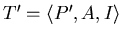must satisfy can be rewritten as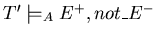, where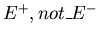stands for the conjunction of all positive examples and of the negation of all negative examples1.

We tried to learn the concept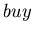. We used as negative examples all the facts for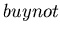. The first experiment was conducted using the information on all available attributes and gave the results: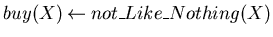113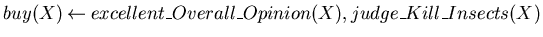22 (18) 0 (74)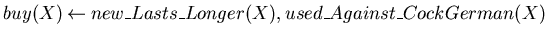21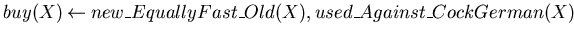9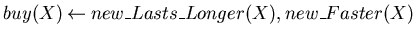6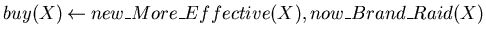5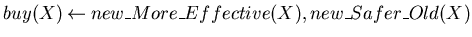4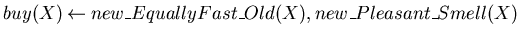4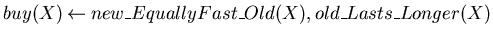1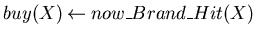1

Rules are followed by a maximum of 4 numbers in this form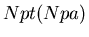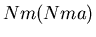.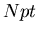is the number of positive examples covered by the rule with or without abduction.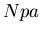is the number of positive examples covered by the rule by using abduction (if absent is 0).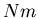is the number of negative examples covered by the rule, i.e.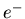for which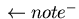failed (if absent is 0, i.e. the rule is consistent).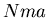is the number of negative examples not covered by using abduction, i.e.succeeded with a non-empty explanation (if absent is 0).

We tested the theory on the 36 examples for don't know buy'' (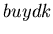). The theory covers all the 36 examples, out of which 13 with abduction.

Then we performed a number of experiments not using part of the background information. We considered the following cases: without the attribute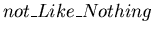, because the most important rule uses only that attribute even if it is not very significative; withoutand the demographic data; using only the predicates that compare the old product with the new one; using only the predicates about the performance on insects.

## Experiments with FOIL

We report the results obtained with the Inductive Logic Programming system FOIL version 6: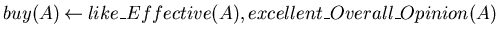123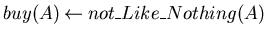23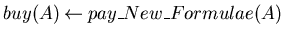6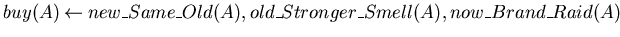5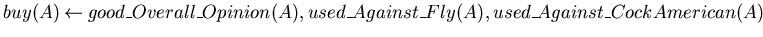5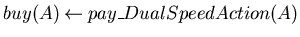2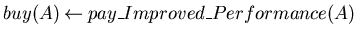1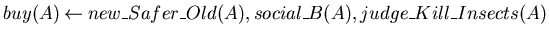2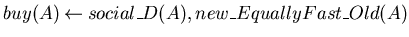1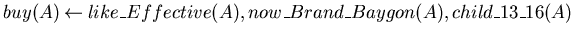2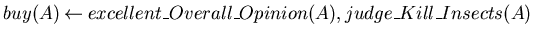1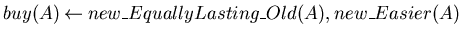1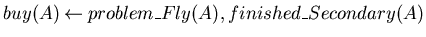1
The figure following the rule represents the number of covered positive examples.

... examples1
It can be shown that this condition is equivalent to that given in the decription of ACL.

back to index